### Sonic in Red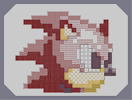Hover over the thumbnail for a full-size version.

Author radioactive_gerbil author:radioactive_gerbil finalstep mines n-art nonplayable rated sonic 2008-02-14 4 by 20 people. \$Sonic in Red#radioactive_gerbil#none#11500000000000000000211150000000000000000000215000000000000000000000200000000000000000000000000000000000000000000000000000000000000000000000000000000000000000000000000000000000000000000000000000000000000000000000000000000000000000000000000000000000000000000000000000000000000000000000000000000000000000000000000000000000000000000000000000000000000000000000000000000000000000000000000000000000000000000000000000000000000000000000000000000000000000000000000000000000000000000000000000000000000000000000000000000000000000000000000000000000000000000000000000000000000000000000000000000000000000000000000000000000000000000000000000000000000000000000400000000000000000000031400000000000000000003111400000000000000000311|7^396,132,1!7^372,156,0!7^372,156,1!7^348,180,0!7^348,204,0!7^348,228,0!7^372,252,3!7^396,252,3!7^420,228,2!7^444,180,1!7^444,180,2!7^420,156,1!7^420,156,2!7^516,252,1!7^468,276,0!7^468,300,0!7^468,324,0!7^540,444,3!7^564,444,3!7^588,444,3!7^612,420,2!7^612,420,3!7^564,300,2!7^564,300,1!7^588,324,2!7^588,324,1!7^612,348,2!7^492,540,0!7^492,540,3!7^468,540,3!7^444,540,3!7^420,516,0!7^420,516,3!7^396,492,0!7^396,492,3!7^372,468,0!7^372,444,0!7^396,420,1!7^396,420,0!7^420,396,1!7^444,396,1!7^468,396,1!7^492,420,2!7^492,420,1!7^636,444,2!7^636,420,2!7^660,372,1!7^636,396,0!7^636,396,1!7^636,420,1!7^636,420,0!7^660,396,1!7^684,420,2!7^684,420,1!7^588,180,0!7^612,204,3!7^612,204,0!7^636,228,3!0^420,420!0^468,444!0^444,444!0^420,444!0^444,468!0^468,492!0^468,468!0^492,468!0^612,444!0^492,492!0^516,492!0^540,492!0^564,492!0^588,468!0^564,468!0^540,468!0^516,468!0^432,432!0^456,456!0^432,456!0^456,480!0^480,480!0^528,480!0^504,480!0^576,480!0^552,480!0^600,456!3^630,426!3^642,426!3^642,414!3^630,414!3^654,402!3^666,402!3^666,390!3^654,390!3^678,426!3^690,426!3^690,414!3^678,414!3^564,324!3^558,324!3^558,318!3^564,318!3^570,318!3^570,324!3^570,330!3^564,330!3^558,330!3^558,336!3^564,336!3^570,336!3^570,342!3^564,342!3^558,342!3^558,348!3^564,348!3^570,348!3^570,354!3^564,354!3^558,354!3^558,360!3^564,360!3^570,360!3^570,366!3^564,366!3^558,366!3^558,372!3^564,372!3^570,372!3^570,378!3^558,378!3^564,378!3^558,384!3^564,384!3^570,384!3^558,390!3^564,390!3^570,390!3^570,396!3^564,396!3^558,396!3^558,402!3^564,402!3^570,402!3^576,402!3^582,402!3^588,402!3^594,402!3^594,396!3^594,390!3^594,342!3^588,342!3^582,342!3^582,348!3^588,348!3^594,348!3^594,354!3^594,360!3^588,360!3^588,354!3^582,354!3^582,360!3^582,366!3^582,372!3^588,372!3^588,366!3^594,366!3^594,372!3^594,378!3^594,384!3^582,384!3^588,384!3^588,378!3^582,378!3^582,390!3^588,390!3^588,396!3^582,396!3^576,396!3^576,390!3^576,384!3^576,378!3^576,372!3^576,366!3^576,360!3^576,354!3^576,348!3^576,342!3^654,414!3^660,414!3^666,414!3^666,420!3^666,426!3^660,426!3^654,426!3^654,420!3^660,420!3^666,432!3^672,438!3^678,438!3^684,438!3^690,438!3^690,444!3^684,444!3^672,444!3^678,444!3^666,444!3^666,438!3^666,432!3^660,432!3^654,432!3^660,438!3^654,438!3^642,438!3^630,438!3^636,438!3^642,438!3^648,438!3^654,444!3^660,444!3^690,450!3^684,450!3^678,450!3^672,450!3^666,450!3^660,450!3^654,450!3^648,450!3^648,444!3^642,444!3^642,450!3^636,450!3^636,444!3^630,444!3^630,450!0^396,156!0^396,180!0^408,192!0^420,180!0^396,204!0^420,204!0^396,444!0^408,444!0^408,456!0^396,456!0^396,468!0^408,468!0^420,468!0^420,492!0^432,492!0^432,480!0^420,480!0^444,492!0^444,504!0^444,516!0^456,516!0^456,504!0^468,504!0^468,516!0^480,516!0^480,504!0^504,504!0^504,516!0^516,516!0^516,504!0^528,504!0^528,516!0^540,516!0^552,516!0^552,504!0^540,504!0^432,408!0^408,408!0^384,432!0^408,432!0^384,456!0^396,480!0^384,480!0^408,480!0^384,468!0^384,444!0^396,432!0^408,492!0^408,504!0^420,504!0^492,504!0^492,516!0^432,504!0^432,516!0^390,438!0^402,438!0^390,450!0^402,450!0^390,462!0^402,462!0^390,474!0^402,474!0^414,486!0^426,486!0^414,474!0^426,498!0^414,498!0^438,498!0^438,510!0^450,510!0^438,522!0^450,522!0^462,522!0^474,522!0^486,522!0^498,522!0^510,522!0^522,522!0^534,522!0^546,522!0^546,510!0^534,510!0^522,510!0^510,510!0^498,510!0^486,510!0^474,510!0^462,510!0^414,414!0^426,414!0^420,408!0^408,420!0^414,426!0^420,432!0^426,426!0^432,420!0^432,528!0^444,528!0^456,528!0^468,528!0^480,528!0^492,528!0^504,528!0^516,528!0^528,528!0^540,528!0^552,528!0^480,432!0^456,432!0^612,468!0^504,552!0^504,540!0^516,540!0^528,540!0^516,552!0^528,552!0^540,540!0^552,540!0^552,552!0^540,552!0^564,552!0^576,552!0^576,540!0^576,528!0^564,528!0^564,540!0^564,504!0^564,516!0^576,516!0^576,504!0^588,504!0^600,504!0^600,516!0^600,528!0^588,528!0^588,516!0^600,492!0^600,480!0^612,480!0^624,480!0^624,492!0^624,504!0^612,504!0^612,492!0^588,492!3^510,534!3^522,534!3^522,546!3^510,546!3^534,546!3^534,534!3^546,534!3^546,546!3^558,546!3^570,546!3^570,534!3^558,534!3^558,522!3^570,522!3^570,510!3^558,510!3^582,510!3^594,510!3^594,522!3^582,522!3^606,498!3^606,486!3^618,486!3^618,498!0^510,534!0^522,534!0^510,546!0^522,546!0^534,546!0^534,534!0^546,534!0^546,546!0^558,546!0^570,546!0^570,534!0^558,534!0^558,522!0^558,510!0^570,510!0^570,522!0^582,522!0^582,510!0^594,510!0^594,522!0^606,498!0^606,486!0^618,486!0^618,498!0^546,498!0^558,498!0^570,498!0^582,498!0^594,498!0^594,486!0^582,486!0^576,492!0^570,486!0^558,486!0^552,492!0^546,486!0^534,498!0^534,486!0^528,492!0^624,456!0^624,432!0^612,432!0^600,432!0^600,420!0^600,408!0^612,408!0^624,408!0^624,420!0^612,420!0^606,414!0^618,414!0^618,426!0^606,426!0^444,420!0^456,408!0^468,420!0^492,444!0^504,456!0^480,456!0^528,456!0^516,444!0^540,444!0^552,432!0^552,456!0^576,456!0^564,444!0^576,432!0^588,444!0^360,168!0^372,168!0^384,168!0^384,216!0^408,216!0^408,228!0^408,240!0^396,240!0^384,240!0^384,228!0^396,228!0^372,228!0^372,240!0^360,240!0^360,228!0^360,216!0^372,216!0^372,204!0^360,204!0^360,192!0^372,192!0^372,180!0^360,180!0^366,174!0^366,186!0^366,198!0^366,210!0^366,222!0^378,222!0^402,234!0^390,234!0^378,234!0^366,234!7^516,396,1!7^540,420,2!0^504,432!0^528,432!7^636,372,2!7^636,396,2!0^384,144!0^408,144!0^408,168!0^432,168!0^432,192!0^456,216!1^588,420!1^564,420!1^540,420!1^540,396!1^516,396!1^516,372!1^492,372!1^492,348!1^492,324!1^492,300!1^492,276!1^516,276!1^516,300!1^516,324!1^516,348!1^540,348!1^540,324!1^540,300!1^540,372!1^612,396!3^636,396!3^642,396!3^642,402!3^636,402!3^630,402!3^630,396!3^630,390!3^636,390!3^642,390!3^642,384!3^642,378!3^636,378!3^630,384!3^636,384!3^630,366!3^636,366!3^642,366!3^642,372!3^636,372!3^648,378!3^648,372!3^648,366!3^654,366!3^660,366!3^666,366!3^666,372!3^666,378!3^660,378!3^660,372!3^654,378!3^654,372!3^660,360!3^666,360!3^654,360!3^654,354!3^660,354!3^666,354!3^666,348!3^660,348!3^654,348!3^654,342!3^660,342!3^666,342!3^666,336!3^660,336!3^654,336!3^654,330!3^660,330!3^666,330!3^666,324!3^660,324!3^654,324!7^468,348,0!12^504,408!12^504,432!12^480,360!12^456,312!12^480,288!12^648,312!12^672,288!12^576,312!12^564,276!12^540,252!12^528,252!12^504,252!12^504,264!12^516,264!12^516,252!12^480,216!12^480,192!12^504,168!12^480,168!12^492,264!12^480,264!12^456,228!12^456,216!12^468,240!12^432,240!7^612,372,2!7^612,372,1!12^600,384!12^612,384!12^624,384!12^624,360!12^600,360!12^612,372!12^600,372!12^480,240!12^480,252!12^468,264!12^492,432!12^480,420!12^480,432!12^504,384!12^480,384!12^486,426!12^492,384!12^504,396!12^456,360!12^468,372!12^480,372!12^456,384!7^468,372,0!7^540,300,3!7^516,276,0!12^528,264!12^456,276!12^480,276!12^468,408!12^462,402!12^456,408!12^456,396!12^588,324!12^582,318!12^594,318!12^594,330!12^582,330!12^600,336!12^588,336!12^576,336!12^576,324!12^600,312!12^606,342!12^618,342!12^624,348!12^624,336!12^600,348!12^648,276!12^654,270!12^666,270!12^672,276!12^672,264!12^660,264!12^648,264!12^624,216!12^630,216!12^636,216!12^642,216!12^648,216!12^648,210!12^648,204!12^648,198!12^648,192!12^642,192!12^636,192!12^630,192!12^624,192!12^624,198!12^624,204!12^624,210!12^630,210!12^636,210!12^642,210!12^642,204!12^642,198!12^636,198!12^630,198!12^630,204!12^636,204!12^624,186!12^630,186!12^636,186!12^642,186!12^648,186!12^648,180!12^642,180!12^636,180!12^630,180!12^624,180!12^618,180!12^618,186!12^618,192!12^612,192!12^606,192!12^600,192!12^600,186!12^606,186!12^612,186!12^612,180!12^606,180!12^600,180!12^600,174!12^606,174!12^612,174!12^618,174!12^624,174!12^630,174!12^636,174!12^642,174!12^648,174!12^648,168!12^648,162!12^648,156!12^648,150!12^648,144!12^648,138!12^648,132!12^648,126!12^648,120!12^636,120!12^642,120!12^630,120!12^624,120!12^624,126!12^624,132!12^630,132!12^630,126!12^636,126!12^642,126!12^642,132!12^636,132!12^636,138!12^642,138!12^642,144!12^642,150!12^642,156!12^642,162!12^642,168!12^636,168!12^630,168!12^624,168!12^618,168!12^612,168!12^606,168!12^600,168!12^600,162!12^600,156!12^600,150!12^600,144!12^606,144!12^612,144!12^618,144!12^624,144!12^624,138!12^630,138!12^630,144!12^636,144!12^636,150!12^636,156!12^636,162!12^630,162!12^624,162!12^618,162!12^612,162!12^606,162!12^606,156!12^606,150!12^612,150!12^618,150!12^624,150!12^630,150!12^630,156!12^624,156!12^618,156!12^612,156!12^624,288!12^624,276!12^636,276!12^624,264!12^636,264!12^432,168!12^438,168!12^444,168!12^450,168!12^456,168!12^456,174!12^456,180!12^456,186!12^450,192!12^444,192!12^438,192!12^432,192!12^432,186!12^432,180!12^432,174!12^444,174!12^450,174!12^450,180!12^444,180!12^444,186!12^450,186!12^438,186!12^438,180!12^438,174!12^426,168!12^420,168!12^414,168!12^408,168!12^408,162!12^408,156!12^408,150!12^408,144!12^414,144!12^420,144!12^432,144!12^432,150!12^432,156!12^432,162!12^426,162!12^420,162!12^414,162!12^414,156!12^414,150!12^420,150!12^426,150!12^426,156!12^420,156!12^402,144!12^396,144!12^390,144!12^384,144!12^384,138!12^384,132!12^384,126!12^384,120!12^396,120!12^390,120!12^402,120!12^408,120!12^408,126!12^408,132!12^408,138!12^402,138!12^396,138!12^390,138!12^390,132!12^390,126!12^396,126!12^402,126!12^402,132!12^396,132!12^384,150!12^384,156!12^384,162!12^384,168!12^378,168!12^372,168!12^366,168!12^360,168!12^360,156!12^360,162!12^360,150!12^360,144!12^360,138!12^360,132!12^360,126!12^360,120!12^366,120!12^372,120!12^378,120!12^378,126!12^378,132!12^378,138!12^378,144!12^378,150!12^378,156!12^378,162!12^372,162!12^366,162!12^366,156!12^366,150!12^366,144!12^366,138!12^366,132!12^366,126!12^372,126!12^372,132!12^372,138!12^372,144!12^372,150!12^372,156!12^360,108!12^360,96!12^372,96!12^384,96!12^384,108!12^372,108!12^396,108!12^408,108!12^408,96!12^396,96!12^420,132!12^420,120!12^432,120!12^432,144!12^432,132!12^444,120!12^444,132!12^444,156!12^426,144!12^444,144!12^456,156!12^456,144!12^456,132!12^456,120!12^468,192!12^468,168!12^468,156!12^468,144!12^480,144!12^480,156!12^492,144!12^504,144!12^516,144!12^516,156!12^504,156!12^528,144!12^528,156!12^516,168!12^528,168!12^468,120!12^480,120!12^480,132!12^468,132!12^528,180!12^540,168!12^552,168!12^552,180!12^564,168!12^576,168!12^576,180!12^576,192!12^564,192!12^564,180!12^576,204!12^588,204!12^612,204!12^600,204!12^600,216!12^588,216!12^600,228!12^612,228!12^612,216!12^588,240!12^600,240!12^612,240!12^624,228!12^624,240!12^636,360!12^648,360!12^648,348!12^648,336!12^636,336!12^636,324!12^648,324!12^636,312!12^624,312!12^612,312!12^624,324!12^600,300!12^612,300!12^624,300!12^612,288!12^600,252!12^612,252!12^624,252!12^420,108!12^432,108!12^444,108!12^456,108!12^468,108!12^480,108!12^480,96!12^468,96!12^456,96!12^444,96!12^432,96!12^420,96!12^414,102!12^414,114!12^426,114!12^426,102!12^438,102!12^450,102!12^462,102!12^474,102!12^474,114!12^462,114!12^450,114!12^438,114!12^492,120!12^504,120!12^516,120!12^528,120!12^528,132!12^516,132!12^504,132!12^492,132!12^486,126!12^498,126!12^510,126!12^522,126!12^522,138!12^510,138!12^498,138!12^486,138!12^540,144!12^552,144!12^564,144!12^576,144!12^576,156!12^564,156!12^552,156!12^540,156!12^534,150!12^546,150!12^558,150!12^570,150!12^570,162!12^558,162!12^546,162!12^534,162!12^582,174!12^594,174!12^594,186!12^582,186!12^588,192!12^588,180!12^588,168!12^606,198!12^618,198!12^618,210!12^606,210!12^630,222!12^642,222!12^636,228!12^648,228!12^648,240!12^636,240!12^630,234!12^642,234!12^630,246!12^642,246!12^642,258!12^630,258!12^648,252!12^636,252!12^480,180!12^468,180!12^492,168!12^492,156!12^540,180!12^564,204!0^432,216!12^444,228!12^432,228!12^432,216!12^420,216!12^420,228!12^420,240!12^456,264!12^444,264!12^648,288!12^636,288!12^636,300!12^648,300!12^576,264!12^612,264!12^600,264!12^588,264!12^612,276!3^666,318!3^660,318!3^654,318!12^396,84!12^390,90!12^402,90!12^408,84!12^408,72!12^402,78!12^396,72!12^390,78!12^384,72!12^384,84!12^378,90!12^366,90!12^372,84!12^378,78!12^366,78!12^372,72!12^360,72!12^360,84!12^354,90!12^354,78!12^348,96!12^348,84!12^348,72!12^342,90!12^342,78!12^336,96!12^336,84!12^336,72!12^330,90!12^330,78!12^324,72!12^324,84!12^324,96!12^318,90!12^318,78!12^312,72!12^312,84!12^312,96!12^306,90!12^306,78!12^300,96!12^300,84!12^300,72!12^294,90!12^294,78!12^288,72!12^288,84!12^288,96!12^486,534!12^498,534!12^480,528!12^492,528!12^504,528!12^492,540!12^498,546!12^504,552!12^492,552!12^480,552!12^486,546!12^480,540!12^474,198!12^480,204!12^456,192!12^468,204!12^462,198!12^462,210!12^474,210!12^468,216!7^468,204,2!0^444,204!12^444,216!7^444,228,3!7^420,228,3!0^396,216!0^390,222!0^402,222!0^378,210!0^378,198!0^378,186!0^378,174!0^384,180!0^384,192!0^384,204!12^588,228!12^588,252!12^564,264!12^612,336!12^636,348!3^630,372!3^630,378!12^612,348!12^606,354!12^618,354!12^612,360!12^624,372!12^612,324!12^600,324!12^588,312!12^576,276!12^588,300!12^576,300!12^576,288!12^564,288!12^474,552!12^474,546!12^474,540!12^474,534!12^474,528!12^468,528!12^462,528!12^456,528!12^456,540!12^456,534!12^462,534!12^468,534!12^468,540!12^462,540!12^462,546!12^468,546!12^468,552!12^462,552!12^456,552!12^456,546!12^450,552!12^450,546!12^450,540!12^450,534!12^450,528!12^444,528!12^438,528!12^432,528!12^432,534!12^432,540!12^432,546!12^432,552!12^438,552!12^444,552!12^444,546!12^444,534!12^438,534!12^438,540!12^438,546!12^444,540!10^378,510!10^372,510!10^366,510!10^366,516!10^366,522!10^372,522!10^378,522!10^378,516!10^372,516!10^426,534!10^420,534!10^414,534!10^408,534!10^402,534!10^396,534!10^390,534!10^390,540!10^390,546!10^396,546!10^402,546!10^408,546!10^414,546!10^420,546!10^426,546!10^426,540!10^420,540!10^414,540!10^408,540!10^402,540!10^396,540!10^384,528!10^384,522!10^384,516!10^384,510!10^384,504!10^378,504!10^366,504!10^372,504!10^360,504!10^360,510!10^360,522!10^360,516!10^360,528!10^366,528!10^372,528!10^378,528!10^426,552!10^414,552!10^420,552!10^408,552!10^402,552!10^396,552!10^390,552!10^384,552!10^384,546!10^384,540!10^384,534!10^426,528!10^420,528!10^414,528!10^402,528!10^396,528!10^390,528!10^552,318!10^558,318!10^564,318!10^570,318!10^576,318!10^552,324!10^552,330!10^552,336!10^552,348!10^552,342!10^552,354!10^552,360!10^552,366!10^552,372!10^552,378!10^552,384!10^552,390!10^552,396!10^552,402!10^552,408!10^558,408!10^564,408!10^570,408!10^576,408!10^582,408!10^588,408!10^594,408!10^600,408!10^600,402!10^600,396!10^600,390!10^600,384!10^600,378!10^600,372!10^600,366!10^600,360!10^600,354!10^600,348!10^600,342!10^594,342!10^588,342!10^582,342!10^576,342!10^576,336!10^576,330!10^576,324!10^654,414!10^660,414!10^666,414!10^666,420!10^666,426!10^666,432!10^666,438!10^672,438!10^678,438!10^684,438!10^690,438!10^690,444!10^690,450!10^684,450!10^678,450!10^672,450!10^666,450!10^660,450!10^654,450!10^648,450!10^642,450!10^636,450!10^630,450!10^630,444!10^630,438!10^636,438!10^642,438!10^648,438!10^654,438!10^654,432!10^654,426!10^654,420!10^642,402!10^636,402!10^630,402!10^630,396!10^630,384!10^630,390!10^630,378!10^630,372!10^630,366!10^636,366!10^642,366!10^648,366!10^654,366!10^654,360!10^654,348!10^654,354!10^654,342!10^654,336!10^654,330!10^654,324!10^654,318!10^660,318!10^666,318!10^666,324!10^666,330!10^666,336!10^666,342!10^666,348!10^666,354!10^666,360!10^666,366!10^666,372!10^666,378!10^660,378!10^654,378!10^648,378!10^642,378!10^642,384!10^642,390!10^642,396!12^552,276!12^552,264!12^540,264!12^552,252!12^468,252!12^492,240!12^492,252!12^516,240!12^504,240!7^492,252,1!12^540,240!12^528,240!12^552,240!12^354,102!12^342,102!12^354,162!12^354,150!12^354,138!12^354,126!12^354,114!12^348,108!12^348,120!12^348,132!12^348,144!12^348,156!12^348,168!12^342,162!12^342,150!12^342,138!12^342,126!12^342,114!12^336,108!12^336,120!12^336,132!12^336,144!12^336,156!12^336,168!12^330,102!12^330,114!12^330,126!12^330,138!12^330,150!12^330,162!12^324,168!12^324,156!12^324,144!12^324,132!12^324,120!12^324,108!12^318,162!12^318,150!12^318,138!12^318,126!12^318,114!12^318,102!12^312,168!12^312,162!12^312,156!12^312,150!12^312,144!12^312,132!12^312,120!12^312,108!12^306,102!12^306,114!12^306,126!12^306,138!12^306,144!12^306,150!12^306,156!12^306,162!12^306,168!12^300,168!12^300,162!12^300,156!12^300,150!12^300,144!12^300,132!12^300,120!12^300,108!12^486,414!12^492,408!12^468,360!12^294,102!12^294,114!12^294,126!12^294,138!12^294,144!12^294,150!12^294,156!12^294,162!12^294,168!12^288,168!12^288,162!12^288,156!12^288,150!12^288,144!12^288,132!12^288,120!12^288,108!12^282,102!12^282,114!12^282,126!12^282,138!12^282,144!12^282,150!12^282,156!12^282,162!12^282,168!12^276,168!12^276,162!12^276,156!12^276,150!12^276,144!12^276,132!12^276,120!12^276,108!12^276,96!12^270,168!12^270,162!12^270,156!12^270,150!12^270,144!12^270,138!12^270,126!12^270,114!12^270,102!12^264,168!12^264,162!12^264,156!12^264,150!12^264,144!12^264,132!12^264,120!12^264,108!12^264,96!12^468,276!12^456,288!12^456,336!12^462,390!12^468,384!12^456,372!12^492,420!12^498,414!12^498,426!12^504,420!12^492,396!12^480,408!12^480,396!12^468,396!12^474,390!12^474,402!7^492,396,3!7^492,396,0!12^258,168!12^258,162!12^258,156!12^258,150!12^258,144!12^258,138!12^258,126!12^258,114!12^258,102!12^252,96!12^252,108!12^252,120!12^252,132!12^252,144!12^252,150!12^252,156!12^252,162!12^252,168!12^246,168!12^246,162!12^246,156!12^246,150!12^246,144!12^246,138!12^246,126!12^246,114!12^246,102!12^240,96!12^240,108!12^240,120!12^240,132!12^240,126!12^240,138!12^240,144!12^240,150!12^240,162!12^240,156!12^240,168!12^234,168!12^234,162!12^234,156!12^234,150!12^234,144!12^234,138!12^234,132!12^234,126!12^234,114!12^234,102!12^228,168!12^228,162!12^228,156!12^228,150!12^228,144!12^228,138!12^228,132!12^228,126!12^228,120!12^228,108!12^228,96!12^222,168!12^222,162!12^222,156!12^222,150!12^222,144!12^222,138!12^222,132!12^222,126!12^222,114!12^222,102!12^216,168!12^216,162!12^216,156!12^216,150!12^216,144!12^216,138!12^216,132!12^216,126!12^216,120!12^216,108!12^216,96!12^210,168!12^210,162!12^210,156!12^210,144!12^210,150!12^210,138!12^210,132!12^210,126!12^210,114!12^210,102!12^204,168!12^204,162!12^204,156!12^204,150!12^204,144!12^204,138!12^204,132!12^204,126!12^204,120!12^204,108!12^204,96!12^198,168!12^198,162!12^198,156!12^198,150!12^198,144!12^198,138!12^198,132!12^198,126!12^198,114!12^198,102!12^192,96!12^192,108!12^192,120!12^192,126!12^192,132!12^192,138!12^192,144!12^192,150!12^192,156!12^192,162!12^192,168!12^186,144!12^186,138!12^186,132!12^186,126!12^186,120!12^180,120!12^180,126!12^180,132!12^180,138!12^180,144!12^174,144!12^174,132!12^174,126!12^174,120!12^168,120!12^168,126!12^168,132!12^168,138!12^168,144!12^174,138!12^162,144!12^162,138!12^162,132!12^162,126!12^162,120!12^156,120!12^156,126!12^156,132!12^234,120!12^222,120!12^210,120!12^198,120!12^156,138!12^156,144!12^150,144!12^150,138!12^150,132!12^150,126!12^150,120!12^144,120!12^144,126!12^144,132!12^144,138!12^144,144!12^264,174!12^264,180!12^264,186!12^264,192!12^270,192!12^276,192!12^282,192!12^288,192!12^288,186!12^282,180!12^288,174!12^288,180!12^282,174!12^276,174!12^270,174!12^270,180!12^270,186!12^276,186!12^282,186!12^276,180!12^294,174!12^300,174!12^306,174!12^312,174!12^312,180!12^306,180!12^300,180!12^294,180!12^294,186!12^300,186!12^306,186!12^312,186!12^312,192!12^306,192!12^300,192!12^294,192!12^288,198!12^294,198!12^300,198!12^306,198!12^312,198!12^312,204!12^312,210!12^312,216!12^306,216!12^300,216!12^294,216!12^288,216!12^288,210!12^288,204!12^294,204!12^300,204!12^306,204!12^306,210!12^300,210!12^294,210!12^318,174!12^324,174!12^330,174!12^336,174!12^342,174!12^348,174!12^354,174!12^360,174!12^360,180!12^360,186!12^360,192!12^360,198!12^360,204!12^360,210!12^360,216!12^354,216!12^348,216!12^342,216!12^336,216!12^336,210!12^336,204!12^336,198!12^330,198!12^324,198!12^318,198!12^318,192!12^318,186!12^318,180!12^324,180!12^330,180!12^336,180!12^342,180!12^348,180!12^354,180!12^354,186!12^354,192!12^354,198!12^354,204!12^354,210!12^348,210!12^342,210!12^342,204!12^348,204!12^348,198!12^342,198!12^342,192!12^348,192!12^348,186!12^342,186!12^336,186!12^330,186!12^324,186!12^324,192!12^330,192!12^336,192!12^468,288!12^456,300!12^456,324!12^456,348!12^336,222!12^336,228!12^336,234!12^336,240!12^342,240!12^348,240!12^354,240!12^360,240!12^360,234!12^360,228!12^360,222!12^354,222!12^348,222!12^342,222!12^342,228!12^342,234!12^348,234!12^354,234!12^354,228!12^348,228!12^450,390!12^444,384!12^438,390!12^432,384!12^432,396!12^432,408!12^444,408!12^444,396!12^438,402!12^450,402!12^444,372!12^432,372!12^432,360!12^444,360!12^444,348!12^432,348!12^432,336!12^444,336!12^444,324!12^432,324!12^432,312!12^444,312!12^444,300!12^432,300!12^432,288!12^444,288!12^444,276!12^432,276!12^432,264!12^432,252!12^420,252!12^408,252!12^396,252!12^384,252!12^384,264!12^396,264!12^408,264!12^420,264!12^420,276!12^408,276!12^396,276!12^384,276!12^372,264!12^372,276!12^360,264!12^360,276!12^360,288!12^372,288!12^384,288!12^408,216!12^408,228!12^408,240!12^396,240!12^384,240!12^372,240!12^396,288!12^408,288!12^420,288!12^360,300!12^372,300!12^384,300!12^360,312!12^372,312!12^384,312!12^384,324!12^396,324!12^396,312!12^408,312!12^408,324!12^408,336!12^396,336!12^384,336!12^396,300!12^408,300!12^420,300!12^420,312!12^420,324!12^420,336!12^420,348!12^384,348!12^384,360!12^396,360!12^408,360!12^408,348!12^396,348!12^420,360!12^384,432!12^396,432!12^408,432!12^402,426!12^390,426!12^384,420!12^396,420!12^408,420!12^402,414!12^390,414!12^384,408!12^396,408!12^666,282!12^654,282!12^660,276!12^660,288!12^390,402!12^384,396!12^390,390!12^384,384!12^390,378!12^384,372!12^390,366!12^402,366!12^414,366!12^426,366!12^420,372!12^408,372!12^396,372!12^402,378!12^414,378!12^426,378!12^420,384!12^408,384!12^396,384!12^402,390!12^414,390!12^426,390!12^420,396!12^408,396!12^396,396!12^402,402!12^414,402!12^426,402!12^408,408!12^420,408!7^636,468,2!12^378,246!12^378,258!12^366,258!12^366,246!12^372,252!12^360,252!12^144,504!12^156,504!12^168,504!12^168,516!12^168,528!12^144,528!12^156,528!12^144,516!12^156,516!12^150,510!12^162,510!12^162,522!12^150,522!12^372,408!12^360,408!12^360,396!12^360,384!12^372,384!12^372,396!12^366,390!12^378,390!12^378,402!12^366,402!12^348,384!12^336,384!12^336,372!12^348,372!12^360,372!12^360,360!12^348,360!12^336,360!12^342,366!12^354,366!12^354,378!12^342,378!12^366,378!12^378,378!12^372,372!12^366,366!12^378,366!12^372,360!12^180,504!12^192,504!12^192,492!12^192,480!12^192,468!12^192,456!12^180,456!12^168,456!12^168,468!12^168,480!12^168,492!12^180,492!12^180,480!12^180,468!12^174,462!12^186,462!12^174,474!12^186,474!12^186,486!12^174,486!12^174,498!12^186,498!12^204,480!12^216,480!12^216,468!12^216,456!12^204,456!12^198,462!12^210,462!12^210,474!12^198,474!12^204,468!12^198,450!12^198,438!12^210,438!12^210,450!12^204,444!12^192,444!12^192,432!12^204,432!12^216,432!12^216,444!12^222,474!12^234,474!12^228,480!12^240,480!12^240,468!12^228,468!12^222,462!12^234,462!12^240,456!12^228,456!12^222,450!12^234,450!12^240,444!12^228,444!12^222,438!12^234,438!12^240,432!12^228,432!12^246,450!12^252,456!12^258,450!12^264,456!12^264,444!12^252,444!12^246,438!12^258,438!12^264,432!12^252,432!12^246,426!12^258,426!12^264,420!12^252,420!12^240,420!12^246,414!12^258,414!12^264,408!12^252,408!12^240,408!12^270,450!12^276,456!12^282,450!12^288,456!12^288,444!12^276,444!12^270,438!12^282,438!12^288,432!12^276,432!12^270,426!12^282,426!12^288,420!12^276,420!12^270,414!12^282,414!12^288,408!12^276,408!12^330,234!12^330,222!12^330,210!12^324,204!12^318,210!12^324,216!12^318,222!12^324,228!12^318,234!12^324,240!12^312,240!12^312,228!12^306,234!12^300,240!12^294,234!12^288,240!12^288,228!12^294,222!12^306,222!12^300,228!12^282,234!12^276,240!12^270,234!12^264,240!12^264,228!12^264,216!12^270,222!12^276,216!12^282,222!12^276,228!12^354,246!12^342,246!12^336,252!12^348,252!12^342,258!12^354,258!12^348,264!12^336,264!12^330,258!12^330,246!12^324,252!12^318,246!12^318,258!12^324,264!12^312,264!12^312,252!12^282,246!12^270,246!12^276,252!12^288,252!12^282,258!12^270,258!12^264,252!12^264,264!12^276,264!12^288,264!12^294,240!12^306,240!12^312,246!12^312,258!12^306,264!12^300,264!12^294,264!12^288,258!12^288,246!12^294,246!12^300,246!12^306,246!12^306,252!12^306,258!12^300,258!12^294,258!12^294,252!12^300,252!12^480,534!12^480,546!12^342,354!12^336,348!12^342,342!12^336,336!12^348,336!12^348,348!12^354,354!12^354,342!12^360,348!12^360,336!12^366,354!12^378,354!12^372,348!12^366,342!12^378,342!12^372,336!12^366,330!12^360,324!12^366,318!12^378,318!12^372,324!12^378,330!12^342,330!12^354,330!12^336,324!12^348,324!12^342,318!12^354,318!12^336,312!12^348,312!12^342,306!12^354,306!12^336,300!12^348,300!12^354,294!12^342,294!12^336,288!12^348,288!12^354,282!12^342,282!12^336,276!12^348,276!12^342,270!12^354,270!12^330,330!12^324,336!12^318,330!12^312,336!12^312,324!12^312,312!12^318,318!12^324,324!12^330,318!12^324,312!12^318,306!12^330,306!12^312,300!12^324,300!12^330,294!12^318,294!12^312,288!12^324,288!12^318,282!12^330,282!12^324,276!12^330,270!12^312,276!12^318,270!12^96,336!12^102,336!12^108,336!12^114,336!12^120,336!12^120,342!12^120,348!12^120,354!12^120,360!12^114,360!12^108,360!12^102,360!12^96,360!12^96,354!12^96,348!12^96,342!12^102,342!12^108,342!12^114,342!12^114,348!12^114,354!12^108,354!12^102,354!12^102,348!12^108,348!12^126,330!12^120,324!12^126,318!12^120,312!12^132,312!12^144,312!12^138,318!12^144,324!12^138,330!12^144,336!12^132,336!12^132,324!12^150,330!12^156,336!12^162,330!12^168,336!12^168,324!12^168,312!12^156,312!12^150,318!12^162,318!12^156,324!12^150,306!12^144,300!12^150,294!12^144,288!12^156,288!12^168,288!12^162,294!12^168,300!12^162,306!12^156,300!12^174,306!12^186,306!12^180,300!12^174,294!12^186,294!12^180,288!12^192,288!12^192,300!12^192,312!12^180,312!12^504,540!12^258,246!12^246,246!12^234,246!12^222,246!12^216,240!12^228,240!12^252,240!12^252,252!12^240,252!12^228,252!12^216,252!12^222,258!12^234,258!12^246,258!12^258,258!12^252,264!12^240,264!12^228,264!12^216,264!12^204,264!12^192,264!12^168,264!12^174,270!12^168,276!12^174,282!12^180,276!12^186,282!12^186,270!12^198,270!12^192,276!12^198,282!12^204,276!12^210,270!12^210,282!12^204,288!12^216,288!12^216,276!12^222,270!12^234,270!12^228,276!12^222,282!12^234,282!12^240,276!12^240,288!12^228,288!12^288,270!12^294,270!12^306,276!12^306,270!12^300,270!12^312,270!12^312,282!12^306,288!12^306,282!12^300,282!12^300,288!12^294,288!12^288,288!12^288,282!12^288,276!12^294,276!12^300,276!12^294,282!12^276,276!12^270,270!12^276,270!12^282,270!12^282,276!12^282,282!12^276,282!12^270,282!12^270,276!12^264,270!12^264,276!12^264,282!12^264,288!12^270,288!12^276,288!12^282,288!12^282,264!12^270,264!12^246,270!12^258,270!12^252,276!12^246,282!12^258,282!12^252,288!12^258,294!12^246,294!12^240,300!12^252,300!12^264,300!12^258,306!12^246,306!12^240,312!12^252,312!12^264,312!12^258,318!12^246,318!12^240,324!12^252,324!12^264,324!12^246,330!12^258,330!12^240,336!12^252,336!12^264,336!12^198,306!12^198,294!12^210,294!12^204,300!12^204,312!12^210,306!12^216,312!12^216,300!12^222,294!12^234,294!12^234,306!12^228,312!12^222,306!12^228,300!12^552,288!12^516,180!7^684,300,2!7^516,444,3!12^126,336!12^138,336!12^150,336!12^162,336!12^168,330!12^174,318!12^168,318!12^174,312!12^186,312!12^198,312!12^210,312!12^222,312!12^234,312!12^240,318!12^240,330!12^246,336!12^258,336!12^264,330!12^264,318!12^264,306!12^264,294!12^312,294!12^312,306!12^312,318!12^312,330!12^318,336!12^330,336!12^336,342!12^336,354!12^336,366!12^336,378!12^342,384!12^354,384!12^360,390!12^360,402!12^366,408!12^378,408!12^384,414!12^384,426!12^384,438!12^384,444!12^384,450!12^384,456!12^384,462!12^384,468!12^384,474!12^384,480!12^390,480!12^396,480!12^408,480!12^402,480!12^408,486!12^408,492!12^408,498!12^408,504!12^414,504!12^420,504!12^426,504!12^432,504!12^432,510!12^432,516!12^432,522!12^378,552!12^372,552!12^366,552!12^360,552!12^354,552!12^348,552!12^342,552!12^336,552!12^330,552!12^324,552!12^318,552!12^312,552!12^306,552!12^300,552!12^294,552!12^288,552!12^282,552!12^276,552!12^270,552!12^264,552!12^264,546!12^264,540!12^264,534!12^264,528!12^126,342!12^132,342!12^132,348!12^126,348!12^126,354!12^132,354!12^132,360!12^126,360!12^138,360!12^144,360!12^144,354!12^138,354!12^138,348!12^138,342!12^144,342!12^144,348!12^150,348!12^150,342!12^156,342!12^156,348!12^156,354!12^150,354!12^150,360!12^156,360!12^162,360!12^168,360!12^168,354!12^162,354!12^162,348!12^162,342!12^168,342!12^168,348!12^180,318!12^186,318!12^192,318!12^198,318!12^204,318!12^210,318!12^216,318!12^222,318!12^228,318!12^234,318!12^234,324!12^234,330!12^234,336!12^228,336!12^228,330!12^228,324!12^222,324!12^222,336!12^222,330!12^216,324!12^210,324!12^204,324!12^198,324!12^192,324!12^186,324!12^180,324!12^174,324!12^174,330!12^180,330!12^186,330!12^192,330!12^198,330!12^204,330!12^210,330!12^216,330!12^216,336!12^210,336!12^204,336!12^192,336!12^198,336!12^186,336!12^180,336!12^174,336!12^174,342!12^174,348!12^174,354!12^174,360!12^180,360!12^186,360!12^186,354!12^180,354!12^180,348!12^180,342!12^186,342!12^186,348!12^192,360!12^192,354!12^192,348!12^192,342!12^198,342!12^198,348!12^198,354!12^198,360!12^204,360!12^204,354!12^204,348!12^204,342!12^210,342!12^210,348!12^210,354!12^210,360!12^216,360!12^216,354!12^216,348!12^216,342!12^270,294!12^276,294!12^282,294!12^288,294!12^294,294!12^300,294!12^306,294!12^306,300!12^300,300!12^294,300!12^288,300!12^282,300!12^276,300!12^270,300!12^270,306!12^276,306!12^282,306!12^288,306!12^294,306!12^300,306!12^306,306!12^306,312!12^300,312!12^288,312!12^294,312!12^282,312!12^276,312!12^270,312!12^270,318!12^276,318!12^282,318!12^288,318!12^294,318!12^300,318!12^306,318!12^180,324!12^270,324!12^276,324!12^282,324!12^288,324!12^294,324!12^300,324!12^306,324!12^306,330!12^300,330!12^294,330!12^288,330!12^282,330!12^276,330!12^270,330!12^270,336!12^276,336!12^282,336!12^288,336!12^294,336!12^300,336!12^306,336!12^258,528!12^252,528!12^246,528!12^240,528!12^234,528!12^228,528!12^222,528!12^216,528!12^210,528!12^198,528!12^204,528!12^192,528!12^186,528!12^180,528!12^174,528!12^168,522!12^168,510!12^174,504!12^186,504!12^192,498!12^192,486!12^198,480!12^210,480!12^222,480!12^234,480!12^240,474!12^246,462!12^240,462!12^246,456!12^258,456!12^270,456!12^282,456!12^288,450!12^288,438!12^288,426!12^288,414!10^408,528!12^426,522!12^420,522!12^414,522!12^408,522!12^402,522!12^396,522!12^390,522!12^390,516!12^390,510!12^390,504!12^390,498!12^384,498!12^378,498!12^372,498!12^366,498!12^360,498!12^354,498!12^354,504!12^354,510!12^354,516!12^354,522!12^354,528!12^354,534!12^360,534!12^366,534!12^372,534!12^378,534!12^378,540!12^378,546!12^282,408!12^270,408!12^264,402!12^264,396!12^264,390!12^264,384!12^252,384!12^258,384!12^246,384!12^240,384!12^234,384!12^228,384!12^222,384!12^216,384!12^216,378!12^216,372!12^216,366!12^222,342!12^228,342!12^240,342!12^234,342!12^246,342!12^252,342!12^258,342!12^264,342!12^270,342!12^276,342!12^282,342!12^288,342!12^294,342!12^300,342!12^306,342!12^312,342!12^318,342!12^324,342!12^330,342!12^222,348!12^228,348!12^234,348!12^240,348!12^246,348!12^252,348!12^264,348!12^258,348!12^270,348!12^276,348!12^282,348!12^288,348!12^294,348!12^300,348!12^306,348!12^312,348!12^318,348!12^330,348!12^324,354!12^324,348!12^330,354!12^222,354!12^228,354!12^234,354!12^240,354!12^246,354!12^252,354!12^258,354!12^264,354!12^270,354!12^276,354!12^282,354!12^288,354!12^294,354!12^300,354!12^306,354!12^312,354!12^318,354!12^222,360!12^228,360!12^234,360!12^240,360!12^246,360!12^252,360!12^258,360!12^264,360!12^270,360!12^276,360!12^282,360!12^288,360!12^294,360!12^300,360!12^312,360!12^306,360!12^318,360!12^324,360!12^330,360!12^222,366!12^228,366!12^234,366!12^240,366!12^246,366!12^252,366!12^258,366!12^264,366!12^270,366!12^276,366!12^282,366!12^288,366!12^294,366!12^300,366!12^306,366!12^312,366!12^318,366!12^324,366!12^330,366!12^222,372!12^228,372!12^234,372!12^240,372!12^246,372!12^252,372!12^258,372!12^264,372!12^270,372!12^276,372!12^282,372!12^288,372!12^294,372!12^300,372!12^306,372!12^312,372!12^318,372!12^324,372!12^330,372!12^222,378!12^228,378!12^240,378!12^234,378!12^246,378!12^252,378!12^258,378!12^264,378!12^270,378!12^276,378!12^282,378!12^288,378!12^294,378!12^300,378!12^306,378!12^312,378!12^318,378!12^324,378!12^330,378!12^270,384!12^276,384!12^282,384!12^288,384!12^294,384!12^300,384!12^306,384!12^312,384!12^318,384!12^324,384!12^330,384!12^426,516!12^426,510!12^420,510!12^420,516!12^414,516!12^414,510!12^408,510!12^408,516!12^402,516!12^396,516!12^396,510!12^402,510!12^402,504!12^396,504!12^396,498!12^402,498!12^402,492!12^402,486!12^396,486!12^396,492!12^390,492!12^390,486!12^384,486!12^384,492!12^378,492!12^270,390!12^276,390!12^282,390!12^288,390!12^294,390!12^300,390!12^306,390!12^312,390!12^318,390!12^324,390!12^330,390!12^336,390!12^342,390!12^348,390!12^354,390!12^270,396!12^276,396!12^282,396!12^288,396!12^294,396!12^300,396!12^306,396!12^312,396!12^318,396!12^324,396!12^330,396!12^336,396!12^342,396!12^348,396!12^354,396!12^270,402!12^282,402!12^276,402!12^288,402!12^294,402!12^306,402!12^300,402!12^312,402!12^318,402!12^324,402!12^330,402!12^336,402!12^342,402!12^348,402!12^354,402!12^294,408!12^300,408!12^306,408!12^318,408!12^312,408!12^324,408!12^330,408!12^336,408!12^342,408!12^348,408!12^354,408!12^294,414!12^300,414!12^306,414!12^312,414!12^318,414!12^324,414!12^330,414!12^336,414!12^342,414!12^348,414!12^354,414!12^360,414!12^372,414!12^366,414!12^378,414!12^294,420!12^300,420!12^306,420!12^312,420!12^318,420!12^324,420!12^336,420!12^330,420!12^342,420!12^348,420!12^354,420!12^360,420!12^366,420!12^372,420!12^378,420!12^294,426!12^300,426!12^306,426!12^312,426!12^318,426!12^324,426!12^330,426!12^336,426!12^342,426!12^348,426!12^354,426!12^360,426!12^366,426!12^372,426!12^378,426!12^282,492!12^294,504!12^312,492!12^342,468!12^336,462!12^312,468!12^330,480!12^330,510!12^318,516!12^330,534!12^318,540!12^300,534!12^282,516!12^252,510!12^228,516!12^204,516!12^186,516!12^216,498!12^240,492!12^258,486!12^282,468!12^300,462!12^312,444!12^330,444!12^360,444!12^360,462!12^360,468!12^366,474!12^354,486!12^342,486!12^342,528!12^360,540!12^276,540!12^312,510!12^282,492!12^300,486!12^318,480!12^342,456!12^366,444!12^348,450!12^348,462!12^318,456!12^318,486!12^306,486!12^324,504!12^300,504!12^300,528!12^312,534!12^312,522!12^300,522!12^270,516!12^270,504!12^270,492!12^270,486!12^270,474!12^264,474!12^252,474!12^252,492!12^264,498!12^246,504!12^240,504!12^216,498!12^234,492!12^228,504!12^216,510!12^210,492!12^204,492!12^204,504!12^366,540!12^372,540!12^372,546!12^366,546!12^354,546!12^360,546!12^354,540!12^348,540!12^348,546!12^342,546!12^336,546!12^330,546!12^324,546!12^312,546!12^318,546!12^306,546!12^300,546!12^294,546!12^282,546!12^288,546!12^276,546!12^270,546!12^270,540!12^282,540!12^288,540!12^294,540!12^300,540!12^312,540!12^306,540!12^324,540!12^330,540!12^336,540!12^342,540!12^336,534!12^342,534!12^348,534!12^270,534!12^276,534!12^282,534!12^288,534!12^294,534!12^306,534!12^318,534!12^324,534!12^348,528!12^336,528!12^330,528!12^324,528!12^318,528!12^312,528!12^306,528!12^294,528!12^288,528!12^282,528!12^276,528!12^270,528!12^174,522!12^180,522!12^186,522!12^192,522!12^198,522!12^204,522!12^210,522!12^222,522!12^216,522!12^228,522!12^234,522!12^240,522!12^246,522!12^252,522!12^258,522!12^264,522!12^270,522!12^276,522!12^282,522!12^288,522!12^294,522!12^306,522!12^318,522!12^324,522!12^330,522!12^336,522!12^342,522!12^348,522!12^348,516!12^342,516!12^336,516!12^330,516!12^324,516!12^312,516!12^300,516!12^306,516!12^294,516!12^288,516!12^276,516!12^264,516!12^258,516!12^252,516!12^246,516!12^240,516!12^234,516!12^222,516!12^216,516!12^210,516!12^198,516!12^192,516!12^180,516!12^174,516!12^174,510!12^180,510!12^186,510!12^192,510!12^204,510!12^198,510!12^210,510!12^222,510!12^228,510!12^234,510!12^240,510!12^246,510!12^258,510!12^264,510!12^270,510!12^276,510!12^282,510!12^294,510!12^288,510!12^300,510!12^306,510!12^318,510!12^324,510!12^336,510!12^342,510!12^348,510!12^378,486!12^378,480!12^378,474!12^378,468!12^378,462!12^378,450!12^378,456!12^378,444!12^378,438!12^378,432!12^204,486!12^198,492!12^198,486!12^198,498!12^198,504!12^204,498!12^210,498!12^210,504!12^216,504!12^222,504!12^222,498!12^222,492!12^216,492!12^216,486!12^210,486!12^222,486!12^228,486!12^228,492!12^228,498!12^234,498!12^234,504!12^240,498!12^246,498!12^252,498!12^252,504!12^258,504!12^264,504!12^258,498!12^246,492!12^234,486!12^240,486!12^246,486!12^252,486!12^252,480!12^246,474!12^246,468!12^252,468!12^246,480!12^252,462!12^258,462!12^264,462!12^270,462!12^270,468!12^264,468!12^258,468!12^258,474!12^258,480!12^264,480!12^264,486!12^264,492!12^258,492!12^270,498!12^270,480!12^276,504!12^282,504!12^288,504!12^288,498!12^282,498!12^276,498!12^276,492!12^276,486!12^276,480!12^276,474!12^276,468!12^276,462!12^282,462!12^288,462!12^288,468!12^288,474!12^282,474!12^282,480!12^282,486!12^288,486!12^288,480!12^288,492!12^294,498!12^300,498!12^306,504!12^312,504!12^318,504!12^330,504!12^336,504!12^342,504!12^348,504!12^348,498!12^342,498!12^330,498!12^336,498!12^324,498!12^312,498!12^318,498!12^306,498!12^306,492!12^300,492!12^294,492!12^294,486!12^294,480!12^294,474!12^294,468!12^294,462!12^294,450!12^294,456!12^294,444!12^294,438!12^294,432!12^300,432!12^300,438!12^300,444!12^300,450!12^300,456!12^300,468!12^300,474!12^300,480!12^306,480!12^306,474!12^306,468!12^306,462!12^306,456!12^306,450!12^306,444!12^306,438!12^306,432!12^312,432!12^312,438!12^312,450!12^312,456!12^312,462!12^312,474!12^312,480!12^312,486!12^318,432!12^324,432!12^330,432!12^336,432!12^342,432!12^348,432!12^354,432!12^360,432!12^372,432!12^366,432!12^372,438!12^366,438!12^360,438!12^354,438!12^348,438!12^342,438!12^336,438!12^330,438!12^324,438!12^318,438!12^318,444!12^324,444!12^324,450!12^318,450!12^318,462!12^318,468!12^318,474!12^318,492!12^372,444!12^354,444!12^348,444!12^342,444!12^336,444!12^342,450!12^336,450!12^330,450!12^336,456!12^330,456!12^324,456!12^324,492!12^324,486!12^324,480!12^324,474!12^324,462!12^324,468!12^330,462!12^330,468!12^330,474!12^330,486!12^330,492!12^336,492!12^342,492!12^348,492!12^354,492!12^360,492!12^366,492!12^372,492!12^336,486!12^348,486!12^360,486!12^366,486!12^372,486!12^372,474!12^372,480!12^372,468!12^372,456!12^372,462!12^372,450!12^366,450!12^360,450!12^354,450!12^336,468!12^336,474!12^336,480!12^342,480!12^348,480!12^360,480!12^354,480!12^366,480!12^366,468!12^366,462!12^366,456!12^360,456!12^354,456!12^348,456!12^342,462!12^354,462!12^354,468!12^354,474!12^348,474!12^342,474!12^348,468!12^360,474!12^180,264!12^240,240# WooSaa! Its finally ready! Well, it was an absolute pain in the arse to finish, over a day to complete from tireful working in between homework and revision! Now I already know from the previous steps that it looks like Knuckles, but I couldnt get the blue shading right! So I stuck to doing it red. And I will make Knuckles soon, maybe Tails as well! RCE :)

## Other maps by this author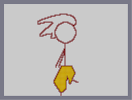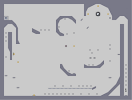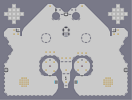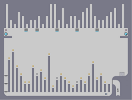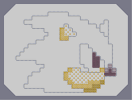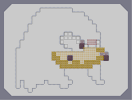The Fancy Pants Man Confusion in an Illusion The Mad Party Contrary to Popular Believe Sonic - Step1 K.T.E - Step1

Pages: (0)

### RED

HOT FUCKING SONIC.

Here it is. =)

### yes dagga

this is the 8 or 16 bit (cant remember which) graphic, which if you look closely is pixelated.

### sonic

is a video game character, therefore he /should/ be pixelated.
he looks a bit too pixeled

### go here

http://numa.notdot.net/map/116317
I found a new (I think) way of making blue! Try it!

5

### This is awesome!

I would recommend getting rid of the bounce blocks in the eyes, but other than that, this is awesome.

### foreword:

knuckles is now in the making!

### The Gerbil Strikes Again!

The sonic map is finished! Big woot!
Faved.
(Do Knuckles next...)